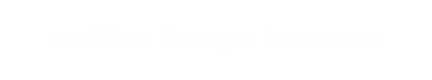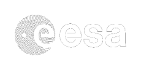# NAVCAM Image Processing Information

This guide provides detailed information for the processing applied to generate the Comet phase images available in this tool. Cruise phase images however have been generated with GDAL as follows.

`gdal_translate -ot byte -scale -of PNG in.IMG out.PNG`

### A. Software & Libraries referred to in this guide

• ImageMagick
• AstroPython libraries & updated python library path, if needed
• python-devel
• python-numpy-devel

The setup referred to below runs as a virtual machine under windows, in that context ~/Win7 refers to a linux mounted windows drive.

## B. Setting up the environment

Download the tarball from: https://pypi.python.org/pypi/astropy and execute as follows:

```tar -xvzf
~/Win7/astropy-0.4.2.tar.gz
cd astropy-0.4.2/
sudo python setup.py
install
cd ..```

### B2. Install specific python packages

You may also need to install python-devel and python-numpy-devel as packages for your distribution.
Under OpenSuse this is:

```sudo zypper install python-devel
sudo zypper install python-numpy-devel```

other Linux packages have their own "zypper" equivalent.

## C. Processing of the images

The steps are as follows:

• C1. Background subtraction
• C2. Intensity scaling
• C3. Vignetting correction
• C4. Pixel pair removal
• C5. 180 degree rotation
• C6. Gamma correction of 1.8

### C1. Background subtraction

Script to be used : bgsub.py

The background subtraction is performed through subtracting an image obtained by selecting the minimum pixel value from a collection of 'average' background images. The minimum pixel value, and not the mean or median was used to avoid contamination from point sources, which otherwise would have to be removed first.

These background images are:

• ROS_CAM1_20140703T090803.FITS
• ROS_CAM1_20140703T091002.FITS
• ROS_CAM1_20140704T103303.FITS
• ROS_CAM1_20140704T103502.FITS
• ROS_CAM1_20140705T085804.FITS
• ROS_CAM1_20140705T090002.FITS
• ROS_CAM1_20140706T085304.FITS
• ROS_CAM1_20140706T085503.FITS
• ROS_CAM1_20140707T084804.FITS
• ROS_CAM1_20140707T085003.FITS
• ROS_CAM1_20140708T084303.FITS
• ROS_CAM1_20140708T084502.FITS
• ROS_CAM1_20140709T073803.FITS
• ROS_CAM1_20140709T074002.FITS

These particular images are not part of the first, but only of the second NAVCAM data release.

### C2. Intensity scaling

Script to be used : bgsub.py

The intensity scaling is performed in order to avoid also scaling on 'empty' pixels. It creates a 2048 channel intensity histogram and then starts adding from channel 0 upwards until it passes 250 pixels (trial and error value). The grey scale associated with that channel is set in all pixels with a value below, such that subsequent ImageMagick intensity scaling is optimised.

### C3. Vignetting correction.

As a one-off, a special vignetting profile is corrected. Note that this does need tuning as the parameters do not always work in the best manner possible. This image is generated with ImageMagick using:

`convert -colorspace gray -size 1024x1024 xc: -monitor -fx "rr=sqrt((i-w/2)^2+(j-h/2)^2); (660/(rr+quantumscale))^8" rolloff.png`

The vignetting itself is used in the processing loop (see full list below) by:

`convert -colorspace gray rolloff.png dif.png -compose divide -composite dif_vc.png`

where dif.png is the PNG generated from the FITS file created in steps c1 and c2.

### C4. Pixel pair removal

Pixel pairs are an effect from radiation damaged pixels which creates two vertically adjacent pixels with one too white and the one vertically next to it too black. This is removed in two identical steps (see full processing below):

```convert -colorspace gray dif_vc.png -morphology Thinning Peaks:1.5 \$f.png
convert -colorspace gray \$f.png +negate tmp.png
convert -colorspace gray tmp.png -morphology Thinning Peaks:1.5 tmp1.png
convert -colorspace gray tmp1.png +negate tmp2.png ```

### C5. 180 degrees rotation

This is performed in order to align the image with the 'true' view generated in our 3d planning tools. Processing is provided in the next step (C6).

### C6. Gamma correction of 1.8

This is performed as it matches the profiles used by many monitors best:

`convert tmp2.png -colorspace gray -quality 100 -rotate 180 -level 0%,100%,1.8 \$f.png`

The full ImageMagick loop (in bash script) - NAVscript.txt - then is:

```
#!/bin/bash
python bgsub.py
mv ROS*.FITS ./Processed
for f in \$MYFILES; do
f_name=\$(basename \$f)
if [ -e ~/Win7/NAVCAM/\$f_name.png ]; then
echo \$f_name' png file already exists'
else
echo \$f_name' new file being processed'
convert -colorspace gray \$f dif.png
convert -colorspace gray rolloff.png dif.png -compose divide -composite dif_vc.png
convert -colorspace gray dif_vc.png -morphology Thinning Peaks:1.5 \$f.png
convert -colorspace gray \$f.png +negate tmp.png
convert -colorspace gray tmp.png -morphology Thinning Peaks:1.5 tmp1.png
convert -colorspace gray tmp1.png +negate tmp2.png
convert tmp2.png -colorspace gray -quality 100 -rotate 180 -level 0%,100%,1.8 \$f.png
mv \$f.png ~/Win7/NAVCAM
rm dif*.png
rm tmp*.png
fi
done
rm *.npy
rm *.FITS
```

bgsub.py is as follows:

```
from astropy.io import fits
from os.path import basename
import glob
import numpy as np

def get_image(filename):

hdulist = fits.open(filename)
image = hdulist
data = np.copy(image.data)
hdulist.close()

return data

def write_matrix_to_FITS(m, name):
hdu = fits.PrimaryHDU(m)
hdulist = fits.HDUList([hdu])
hdulist.writeto(name, clobber=True)

filenames = glob.glob("./FlatField/ROS*.FITS")

print filenames

stack = np.array([ get_image(f) for f in filenames ])

print stack.shape

minimum = np.min(stack, axis=0)
np.save("minimum", minimum)

write_matrix_to_FITS(minimum, 'minimum.FITS')

li = ['000','001','002','003','004','005','006','007','008','009',
'010','011','012','013','014','015','016','017','018','019',
'020','021','022','023','024','025','026','027','028','029',
'030','031','032','033','034','035','036','037','038','039',
'040','041','042']

for s in li:
filenames = glob.glob("./Win7/NAVCAM/STP%s/*.FITS" % (s) )
for f in filenames:
fitsimage = np.array( get_image(f) )
if fitsimage.shape == 1024:
bgsub = fitsimage - minimum
imghist, histedge = np.histogram(bgsub, bins=2048)
imgcum  = np.cumsum(imghist)
counter = 0
while imgcum[counter] < 250:
counter += 1
if imgcum[counter] > 1000:
counter -= 1
if counter < 0:
counter = 0
print f, counter
bgsub[ bgsub < int(histedge[counter]) ] = int(histedge[counter])
write_matrix_to_FITS(bgsub, basename(f))
```